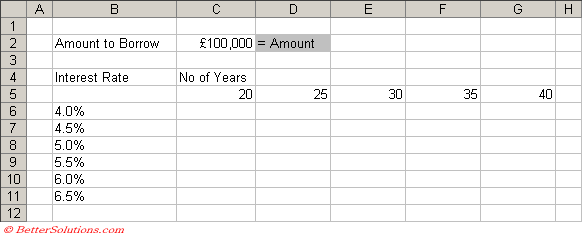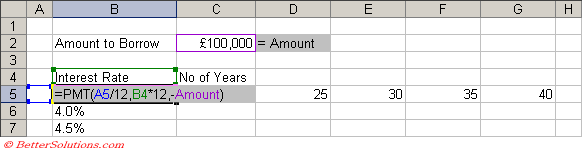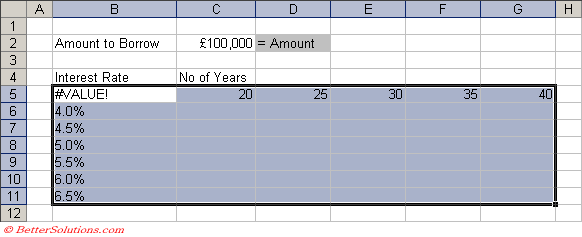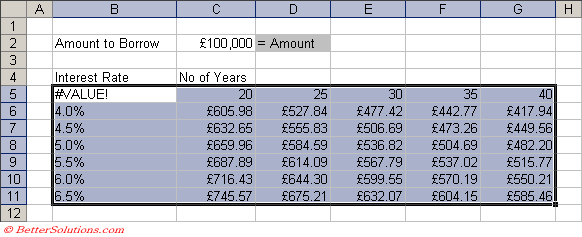# Two Variable

A data table can also summarise the impact of two variables on a formula.
Lets consider the same mortgage calculation but lets suppose that you want to vary the number of years as well as the interest rate.
You can now work out the monthly repayments given a fixed interest rate and a fixed number of years.
This type of problem can be solved by using a two variable data table.

### Create a Simple Table

You must create a table that contains at least two columns and two rows.
The first column should contain the values for one of the arguments you want to substitute in the formula.
The first row should contain the values for the other argument you want to substitute in the formula.
Create a table that displays various interest rates down the left hand side and various years across the top.
The worksheet function that can be used to calculate the payment for a loan given a fixed interest rate is the PMT function.
Create the following table and the following named ranges:
"C2" = Amount### Entering the Formula

The formula you are using must be entered in the cell which is the intersection of the column and row variables. This is cell "B5".
For the data table to work correctly the formula must refer to the cell above for the column variable and the cell to its left for the row variable.
This formula will refer to cell "B4" for the number of years and cell "A5" for the interest rate.
Because the formula does not refer to any cells in the "actual" table it will probably return an error (or at least a meaningless result).
This error (or incorrect result) will not affect the results of the table.
Enter the following formula to calculate the monthly repayment into cell "B5"," =PMT(A5/12,B4*12,-Amount)".### Using Data Table

Highlight the whole table, so in this case "B5:G11".
You must include the whole table including all the input variables.
You do not need to include any column or row headings.Select (Data > Table) to display the Table dialog box.
Enter the cell that corresponds to the first row variable into the "Row input cell". In this case "B4".
Enter the cell that corresponds to the first column variable into the "Column input cell". In this case "A5".Press OK and the table will be automatically populated using the formula provided.You can obviously see that the lower the interest rate and the longer the time span, the lower the monthly repayment.

### Important

The data table has one advantage over a pivot table and that is that it is updated automatically and does not need to be refreshed.